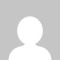Knowledgebase
KnowledgebaseWhat arithmetical and logical operations can I use while editing my templates? Posted by Maksim Karganov (AIT) on 03 June 2011 05:55 PM You can use the following operations: Arithmetical operations: >, <, =, <=, >=, <> (not equal), != (not equal), +, -, *, /, % (modulo) Logical operations: && (and), || (or), ! (not)(47 vote(s))HelpfulNot helpful